·  Page d'accueil  ·  Toutes les classes  ·  Classes principales  ·  Annotées  ·  Classes groupées  ·  Modules  ·  Fonctions  ·

# QPainterPath Class Reference[QtGui module]

The QPainterPath class provides a container for painting operations, enabling graphical shapes to be constructed and reused. More...

` #include <QPainterPath>`

### Public Types

• class Element
• enum ElementType { MoveToElement, LineToElement, CurveToElement, CurveToDataElement }

### Public Functions

• QPainterPath ( const QPointF & startPoint )
• QPainterPath ( const QPainterPath & path )
• void addEllipse ( const QRectF & boundingRectangle )
• void addEllipse ( qreal x, qreal y, qreal width, qreal height )
• void addPath ( const QPainterPath & path )
• void addPolygon ( const QPolygonF & polygon )
• void addRect ( const QRectF & rectangle )
• void addRect ( qreal x, qreal y, qreal width, qreal height )
• void addRegion ( const QRegion & region )
• void addText ( const QPointF & point, const QFont & font, const QString & text )
• void addText ( qreal x, qreal y, const QFont & font, const QString & text )
• void arcMoveTo ( const QRectF & rectangle, qreal angle )
• void arcMoveTo ( qreal x, qreal y, qreal width, qreal height, qreal angle )
• void arcTo ( const QRectF & rectangle, qreal startAngle, qreal sweepLength )
• void arcTo ( qreal x, qreal y, qreal width, qreal height, qreal startAngle, qreal sweepLength )
• QRectF boundingRect () const
• void closeSubpath ()
• void connectPath ( const QPainterPath & path )
• bool contains ( const QPointF & point ) const
• bool contains ( const QRectF & rectangle ) const
• QRectF controlPointRect () const
• void cubicTo ( const QPointF & c1, const QPointF & c2, const QPointF & endPoint )
• void cubicTo ( qreal c1X, qreal c1Y, qreal c2X, qreal c2Y, qreal endPointX, qreal endPointY )
• QPointF currentPosition () const
• const QPainterPath::Element & elementAt ( int index ) const
• int elementCount () const
• Qt::FillRule fillRule () const
• bool intersects ( const QRectF & rectangle ) const
• bool isEmpty () const
• void lineTo ( const QPointF & endPoint )
• void lineTo ( qreal x, qreal y )
• void moveTo ( const QPointF & point )
• void moveTo ( qreal x, qreal y )
• void quadTo ( const QPointF & c, const QPointF & endPoint )
• void quadTo ( qreal cx, qreal cy, qreal endPointX, qreal endPointY )
• void setElementPositionAt ( int index, qreal x, qreal y )
• void setFillRule ( Qt::FillRule fillRule )
• QPolygonF toFillPolygon ( const QMatrix & matrix = QMatrix() ) const
• QList<QPolygonF> toFillPolygons ( const QMatrix & matrix = QMatrix() ) const
• QPainterPath toReversed () const
• QList<QPolygonF> toSubpathPolygons ( const QMatrix & matrix = QMatrix() ) const
• bool operator!= ( const QPainterPath & path ) const
• QPainterPath & operator= ( const QPainterPath & path )
• bool operator== ( const QPainterPath & path ) const

### Related Non-Members

• QDataStream & operator<< ( QDataStream & stream, const QPainterPath & path )
• QDataStream & operator>> ( QDataStream & stream, QPainterPath & path )

## Detailed Description

The QPainterPath class provides a container for painting operations, enabling graphical shapes to be constructed and reused.

A painter path is an object composed of a number of graphical building blocks, such as rectangles, ellipses, lines, and curves. Building blocks can be joined in closed subpaths, for example as a rectangle or an ellipse. A closed path has coinciding start and end points. Or they can exist independently as unclosed subpaths, such as lines and curves.

A QPainterPath object can be used for filling, outlining, and clipping. To generate fillable outlines for a given painter path, use the QPainterPathStroker class. The main advantage of painter paths over normal drawing operations is that complex shapes only need to be created once; then they can be drawn many times using only calls to the QPainter::drawPath() function.

QPainterPath provides a collection of functions that can be used to obtain information about the path and its elements. In addition it is possible to reverse the order of the elements using the toReversed() function. There are also several functions to convert this painter path object into a polygon representation.

### Composing a QPainterPath

A QPainterPath object can be constructed as an empty path, with a given start point, or as a copy of another QPainterPath object. Once created, lines and curves can be added to the path using the lineTo(), arcTo(), cubicTo() and quadTo() functions. The lines and curves stretch from the currentPosition() to the position passed as argument.

The currentPosition() of the QPainterPath object is always the end position of the last subpath that was added (or the initial start point). Use the moveTo() function to move the currentPosition() without adding a component. The moveTo() function implicitly starts a new subpath, and closes the previous one. Another way of starting a new subpath is to call the closeSubpath() function which closes the current path by adding a line from the currentPosition() back to the path's start position. Note that the new path will have (0, 0) as its initial currentPosition().

In addition, a path can be added to the current path using the connectPath() function. But note that this function will connect the last element of the current path to the first element of given one by adding a line.

Below is a code snippet that shows how a QPainterPath object can be used:``` QPainterPath path; path.addRect(20, 20, 60, 60); path.moveTo(0, 0); path.cubicTo(99, 0, 50, 50, 99, 99); path.cubicTo(0, 99, 50, 50, 0, 0); QPainter painter(this); painter.fillRect(0, 0, 100, 100, Qt::white); painter.setPen(QPen(QColor(79, 106, 25), 1, Qt::SolidLine, Qt::FlatCap, Qt::MiterJoin)); painter.setBrush(QColor(122, 163, 39)); painter.drawPath(path);```

The painter path is initially empty when constructed. We first add a rectangle, which is a closed subpath. Then we add two bezier curves which together form a closed subpath even though they are not closed individually. Finally we draw the entire path. The path is filled using the default fill rule, Qt::OddEvenFill. Qt provides two methods for filling paths:

Qt::OddEvenFill Qt::WindingFill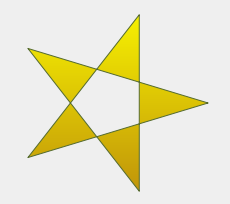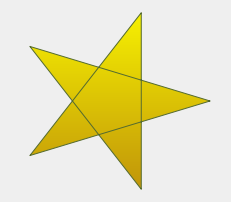See the Qt::Fillrule documentation for the definition of the rules. A painter path's currently set fill rule can be retrieved using the fillRule() function, and altered using the setFillRule() function.

### QPainterPath Information

The QPainterPath class provides a collection of functions that returns information about the path and its elements.

The currentPosition() function returns the end point of the last subpath that was added (or the initial start point). The elementAt() function can be used to retrieve the various subpath elements, the number of elements can be retrieved using the elementCount() function, and the isEmpty() function tells whether this QPainterPath object contains any elements at all.

The controlPointRect() function returns the rectangle containing all the points and control points in this path. This function is significantly faster to compute than the exact boundingRect() which returns the bounding rectangle of this painter path with floating point precision.

Finally, QPainterPath provides the contains() function which can be used to determine whether a given point or rectangle is inside the path, and the intersects() function which determines if any of the points inside a given rectangle also are inside this path.

### QPainterPath Conversion

For compatibility reasons, it might be required to simplify the representation of a painter path: QPainterPath provides the toFillPolygon(), toFillPolygons() and toSubpathPolygons() functions which convert the painter path into a polygon. The toFillPolygon() returns the painter path as one single polygon, while the two latter functions return a list of polygons.

The toFillPolygons() and toSubpathPolygons() functions are provided because it is usually faster to draw several small polygons than to draw one large polygon, even though the total number of points drawn is the same. The difference between the two is the number of polygons they return: The toSubpathPolygons() creates one polygon for each subpath regardless of intersecting subpaths (i.e. overlapping bounding rectangles), while the toFillPolygons() functions creates only one polygon for overlapping subpaths.

The toFillPolygon() and toFillPolygons() functions first convert all the subpaths to polygons, then uses a rewinding technique to make sure that overlapping subpaths can be filled using the correct fill rule. Note that rewinding inserts additional lines in the polygon so the outline of the fill polygon does not match the outline of the path.

### Examples

Qt provides the Painter Paths Example and the Vector Deformation Demo which are located in Qt's example and demo directories respectively.

The Painter Paths Example shows how painter paths can be used to build complex shapes for rendering and lets the user experiment with the filling and stroking. The Vector Deformation Demo shows how to use QPainterPath to draw text.

Painter Paths Example Vector Deformation Demo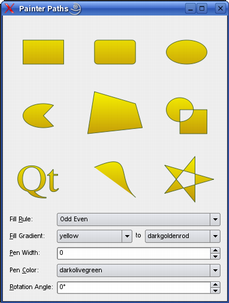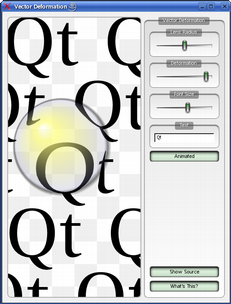## Member Type Documentation

### enum QPainterPath::ElementType

This enum describes the types of elements used to connect vertices in subpaths.

ConstantValueDescription
QPainterPath::CurveToDataElement3The extra data required to describe a curve in a CurveToElement element.

## Member Function Documentation

### QPainterPath::QPainterPath ()

Constructs an empty QPainterPath object.

### QPainterPath::QPainterPath ( const QPointF & startPoint )

Creates a QPainterPath object with the given startPoint as its current position.

### QPainterPath::QPainterPath ( const QPainterPath & path )

Creates a QPainterPath object that is a copy of the given path.

### QPainterPath::~QPainterPath ()

Destroys this QPainterPath object.

### void QPainterPath::addEllipse ( const QRectF & boundingRectangle )

Creates an ellipse within the the specified boundingRectangle and adds it to the painter path as a closed subpath.

The ellipse is composed of a clockwise curve, starting and finishing at zero degrees (the 3 o'clock position).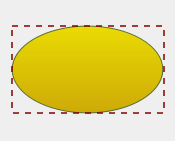``` QLinearGradient myGradient; QPen myPen; QRectF boundingRectangle; QPainterPath myPath; myPath.addEllipse(boundingRectangle); QPainter painter(this); painter.setBrush(myGradient); painter.setPen(myPen); painter.drawPath(myPath);```

### void QPainterPath::addEllipse ( qreal x, qreal y, qreal width, qreal height )

This is an overloaded member function, provided for convenience.

Creates an ellipse within the bounding rectangle defined by its top-left corner at (x, y), width and height, and adds it to the painter path as a closed subpath.

### void QPainterPath::addPath ( const QPainterPath & path )

Adds the given path to this path as a closed subpath.

### void QPainterPath::addPolygon ( const QPolygonF & polygon )

Adds the given polygon to the path as an (unclosed) subpath.

Note that the current position after the polygon has been added, is the last point in polygon. To draw a line back to the first point, use the closeSubpath() function.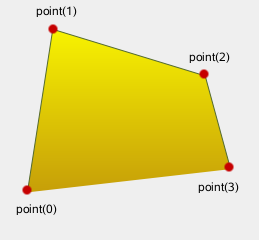``` QLinearGradient myGradient; QPen myPen; QPolygonF myPolygon; QPainterPath myPath; myPath.addPolygon(myPolygon); QPainter painter(this); painter.setBrush(myGradient); painter.setPen(myPen); painter.drawPath(myPath);```

### void QPainterPath::addRect ( const QRectF & rectangle )

Adds the given rectangle to this path as a closed subpath.

The rectangle is added as a clockwise set of lines. The painter path's current position after the rectangle has been added is at the top-left corner of the rectangle.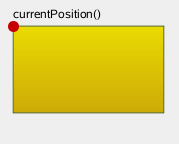``` QLinearGradient myGradient; QPen myPen; QRectF myRectangle; QPainterPath myPath; myPath.addRect(myRectangle); QPainter painter(this); painter.setBrush(myGradient); painter.setPen(myPen); painter.drawPath(myPath);```

### void QPainterPath::addRect ( qreal x, qreal y, qreal width, qreal height )

This is an overloaded member function, provided for convenience.

Adds a rectangle at position (x, y), with the given width and height, as a closed subpath.

### void QPainterPath::addRegion ( const QRegion & region )

Adds the given region to the path by adding each rectangle in the region as a separate closed subpath.

### void QPainterPath::addText ( const QPointF & point, const QFont & font, const QString & text )

Adds the given text to this path as a set of closed subpaths created from the font supplied. The subpaths are positioned so that the left end of the text's baseline lies at the specified point.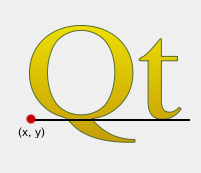``` QLinearGradient myGradient; QPen myPen; QFont myFont; QPointF baseline(x, y); QPainterPath myPath; myPath.addText(baseline, myFont, tr("Qt")); QPainter painter(this); painter.setBrush(myGradient); painter.setPen(myPen); painter.drawPath(myPath);```

### void QPainterPath::addText ( qreal x, qreal y, const QFont & font, const QString & text )

This is an overloaded member function, provided for convenience.

Adds the given text to this path as a set of closed subpaths created from the font supplied. The subpaths are positioned so that the left end of the text's baseline lies at the point specified by (x, y).

### void QPainterPath::arcMoveTo ( const QRectF & rectangle, qreal angle )

Creates a move to that lies on the arc that occupies the given rectangle at angle.

Angles are specified in degrees. Clockwise arcs can be specified using negative angles.

This function was introduced in Qt 4.2.

### void QPainterPath::arcMoveTo ( qreal x, qreal y, qreal width, qreal height, qreal angle )

This is an overloaded member function, provided for convenience.

Creates a move to that lies on the arc that occupies the QRectF(x, y, width, height) at angle.

This function was introduced in Qt 4.2.

### void QPainterPath::arcTo ( const QRectF & rectangle, qreal startAngle, qreal sweepLength )

Creates an arc that occupies the given rectangle, beginning at the specified startAngle and extending sweepLength degrees counter-clockwise.

Angles are specified in degrees. Clockwise arcs can be specified using negative angles.

Note that this function connects the starting point of the arc to the current position if they are not already connected. After the arc has been added, the current position is the last point in arc. To draw a line back to the first point, use the closeSubpath() function.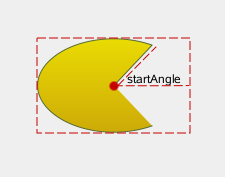``` QLinearGradient myGradient; QPen myPen; QPointF center, startPoint; QPainterPath myPath; myPath.moveTo(center); myPath.arcTo(boundingRect, startAngle, sweepLength); QPainter painter(this); painter.setBrush(myGradient); painter.setPen(myPen); painter.drawPath(myPath);```

### void QPainterPath::arcTo ( qreal x, qreal y, qreal width, qreal height, qreal startAngle, qreal sweepLength )

This is an overloaded member function, provided for convenience.

Creates an arc that occupies the rectangle QRectF(x, y, width, height), beginning at the specified startAngle and extending sweepLength degrees counter-clockwise.

### QRectF QPainterPath::boundingRect () const

Returns the bounding rectangle of this painter path as a rectangle with floating point precision.

### void QPainterPath::closeSubpath ()

Closes the current subpath by drawing a line to the beginning of the subpath, automatically starting a new path. The current point of the new path is (0, 0).

If the subpath does not contain any elements, this function does nothing.

### void QPainterPath::connectPath ( const QPainterPath & path )

Connects the given path to this path by adding a line from the last element of this path to the first element of the given path.

### bool QPainterPath::contains ( const QPointF & point ) const

Returns true if the given point is inside the path, otherwise returns false.

### bool QPainterPath::contains ( const QRectF & rectangle ) const

This is an overloaded member function, provided for convenience.

Returns true if the given rectangle is inside the path, otherwise returns false.

### QRectF QPainterPath::controlPointRect () const

Returns the rectangle containing all the points and control points in this path.

This function is significantly faster to compute than the exact boundingRect(), and the returned rectangle is always a superset of the rectangle returned by boundingRect().

### void QPainterPath::cubicTo ( const QPointF & c1, const QPointF & c2, const QPointF & endPoint )

Adds a cubic Bezier curve between the current position and the given endPoint using the control points specified by c1, and c2.

After the curve is added, the current position is updated to be at the end point of the curve.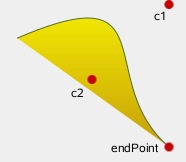``` QLinearGradient myGradient; QPen myPen; QPainterPath myPath; myPath.cubicto(c1, c2, endPoint); QPainter painter(this); painter.setBrush(myGradient); painter.setPen(myPen); painter.drawPath(myPath);```

### void QPainterPath::cubicTo ( qreal c1X, qreal c1Y, qreal c2X, qreal c2Y, qreal endPointX, qreal endPointY )

This is an overloaded member function, provided for convenience.

Adds a cubic Bezier curve between the current position and the end point (endPointX, endPointY) with control points specified by (c1X, c1Y) and (c2X, c2Y).

### QPointF QPainterPath::currentPosition () const

Returns the current position of the path.

### const QPainterPath::Element & QPainterPath::elementAt ( int index ) const

Returns the element at the given index in the painter path.

### int QPainterPath::elementCount () const

Returns the number of path elements in the painter path.

### Qt::FillRule QPainterPath::fillRule () const

Returns the painter path's currently set fill rule.

### bool QPainterPath::intersects ( const QRectF & rectangle ) const

Returns true if any point in the given rectangle intersects the path; otherwise returns false.

There is an intersection if any of the lines making up the rectangle crosses a part of the path or if any part of the rectangle overlaps with any area enclosed by the path. This function respects the current fillRule to determine what is considered inside the path.

### bool QPainterPath::isEmpty () const

Returns true if there are no elements in this path, otherwise returns false.

### void QPainterPath::lineTo ( const QPointF & endPoint )

Adds a straight line from the current position to the given endPoint. After the line is drawn, the current position is updated to be at the end point of the line.

### void QPainterPath::lineTo ( qreal x, qreal y )

This is an overloaded member function, provided for convenience.

Draws a line from the current position to the point (x, y).

### void QPainterPath::moveTo ( const QPointF & point )

Moves the current point to the given point, implicitly starting a new subpath and closing the previous one.

### void QPainterPath::moveTo ( qreal x, qreal y )

This is an overloaded member function, provided for convenience.

Moves the current position to (x, y) and starts a new subpath, implicitly closing the previous path.

### void QPainterPath::quadTo ( const QPointF & c, const QPointF & endPoint )

Adds a quadratic Bezier curve between the current position and the given endPoint with the control point specified by c.

After the curve is added, the current point is updated to be at the end point of the curve.

### void QPainterPath::quadTo ( qreal cx, qreal cy, qreal endPointX, qreal endPointY )

This is an overloaded member function, provided for convenience.

Adds a quadratic Bezier curve between the current point and the endpoint (endPointX, endPointY) with the control point specified by (cx, cy).

### void QPainterPath::setElementPositionAt ( int index, qreal x, qreal y )

Sets the x and y coordinate of the element at index index to x and y.

This function was introduced in Qt 4.2.

### void QPainterPath::setFillRule ( Qt::FillRule fillRule )

Sets the fill rule of the painter path to the given fillRule. Qt provides two methods for filling paths:

Qt::OddEvenFill (default) Qt::WindingFill### QPolygonF QPainterPath::toFillPolygon ( const QMatrix & matrix = QMatrix() ) const

Converts the path into a polygon using the given transformation matrix, and returns the polygon.

The polygon is created by first converting all subpaths to polygons, then using a rewinding technique to make sure that overlapping subpaths can be filled using the correct fill rule.

Note that rewinding inserts addition lines in the polygon so the outline of the fill polygon does not match the outline of the path.

### QList<QPolygonF> QPainterPath::toFillPolygons ( const QMatrix & matrix = QMatrix() ) const

Converts the path into a list of polygons using the given transformation matrix, and returns the list.

The function differs from the toFillPolygon() function in that it creates several polygons. It is provided because it is usually faster to draw several small polygons than to draw one large polygon, even though the total number of points drawn is the same.

The toFillPolygons() function differs from the toSubpathPolygons() function in that it create only polygon for subpaths that have overlapping bounding rectangles.

Like the toFillPolygon() function, this function uses a rewinding technique to make sure that overlapping subpaths can be filled using the correct fill rule. Note that rewinding inserts addition lines in the polygons so the outline of the fill polygon does not match the outline of the path.

### QPainterPath QPainterPath::toReversed () const

Creates and returns a reversed copy of the path.

It is the order of the elements that is reversed: If a QPainterPath is composed by calling the moveTo(), lineTo() and cubicTo() functions in the specified order, the reversed copy is composed by calling cubicTo(), lineTo() and moveTo().

### QList<QPolygonF> QPainterPath::toSubpathPolygons ( const QMatrix & matrix = QMatrix() ) const

Converts the path into a list of polygons using the given transformation matrix, and returns the list.

This function creates one polygon for each subpath regardless of intersecting subpaths (i.e. overlapping bounding rectangles). To make sure that such overlapping subpaths are filled correctly, use the toFillPolygons() function instead.

### bool QPainterPath::operator!= ( const QPainterPath & path ) const

Returns true if this painter path differs from the given path.

Note that comparing paths may involve a per element comparison which can be slow for complex paths.

### QPainterPath & QPainterPath::operator= ( const QPainterPath & path )

Assigns the given path to this painter path.

### bool QPainterPath::operator== ( const QPainterPath & path ) const

Returns true if this painterpath is equal to the given path.

Note that comparing paths may involve a per element comparison which can be slow for complex paths.

## Related Non-Members

### QDataStream & operator<< ( QDataStream & stream, const QPainterPath & path )

This is an overloaded member function, provided for convenience.

Writes the given painter path to the given stream, and returns a reference to the stream.

### QDataStream & operator>> ( QDataStream & stream, QPainterPath & path )

This is an overloaded member function, provided for convenience.

Reads a painter path from the given stream into the specified path, and returns a reference to the stream.

Publicité

## Best Of

Semaine
Mois
Année

### Le blog Digia au hasard#### Déploiement d'applications Qt Commercial sur les tablettes Windows 8

Le blog Digia est l'endroit privilégié pour la communication sur l'édition commerciale de Qt, où des réponses publiques sont apportées aux questions les plus posées au support. Lire l'article.

### Contact

• Vous souhaitez rejoindre la rédaction ou proposer un tutoriel, une traduction, une question... ? Postez dans le forum Contribuez ou contactez-nous par MP ou par email (voir en bas de page).

### Qt dans le magazine

 Cette page est une traduction d'une page de la documentation de Qt, écrite par Nokia Corporation and/or its subsidiary(-ies). Les éventuels problèmes résultant d'une mauvaise traduction ne sont pas imputables à Nokia. Qt 4.2 Copyright © 2012 Developpez LLC. Tous droits réservés Developpez LLC. Aucune reproduction, même partielle, ne peut être faite de ce site et de l'ensemble de son contenu : textes, documents et images sans l'autorisation expresse de Developpez LLC. Sinon, vous encourez selon la loi jusqu'à 3 ans de prison et jusqu'à 300 000 E de dommages et intérêts. Cette page est déposée à la SACD. Vous avez déniché une erreur ? Un bug ? Une redirection cassée ? Ou tout autre problème, quel qu'il soit ? Ou bien vous désirez participer à ce projet de traduction ? N'hésitez pas à nous contacter ou par MP !

Partenaires

Hébergement Web